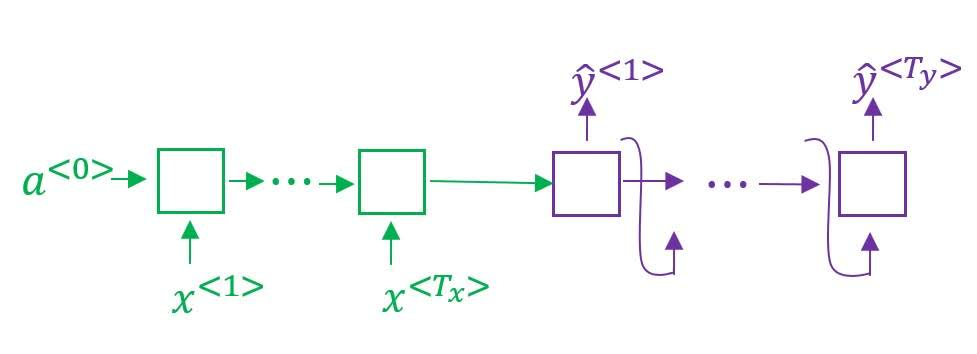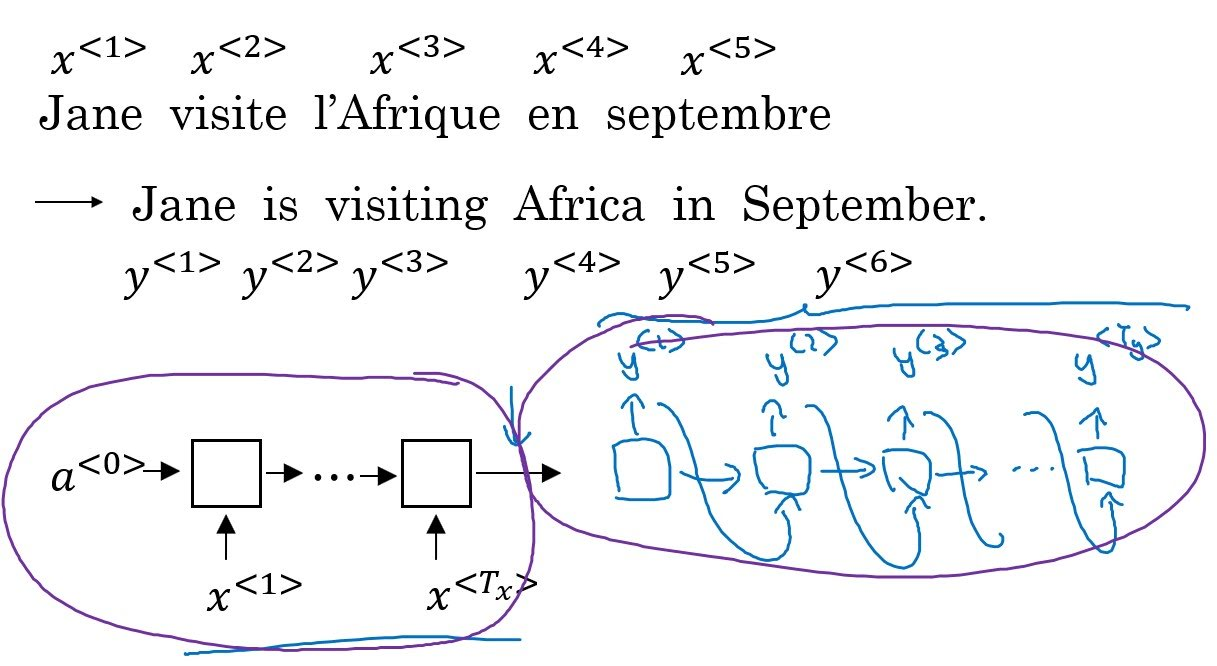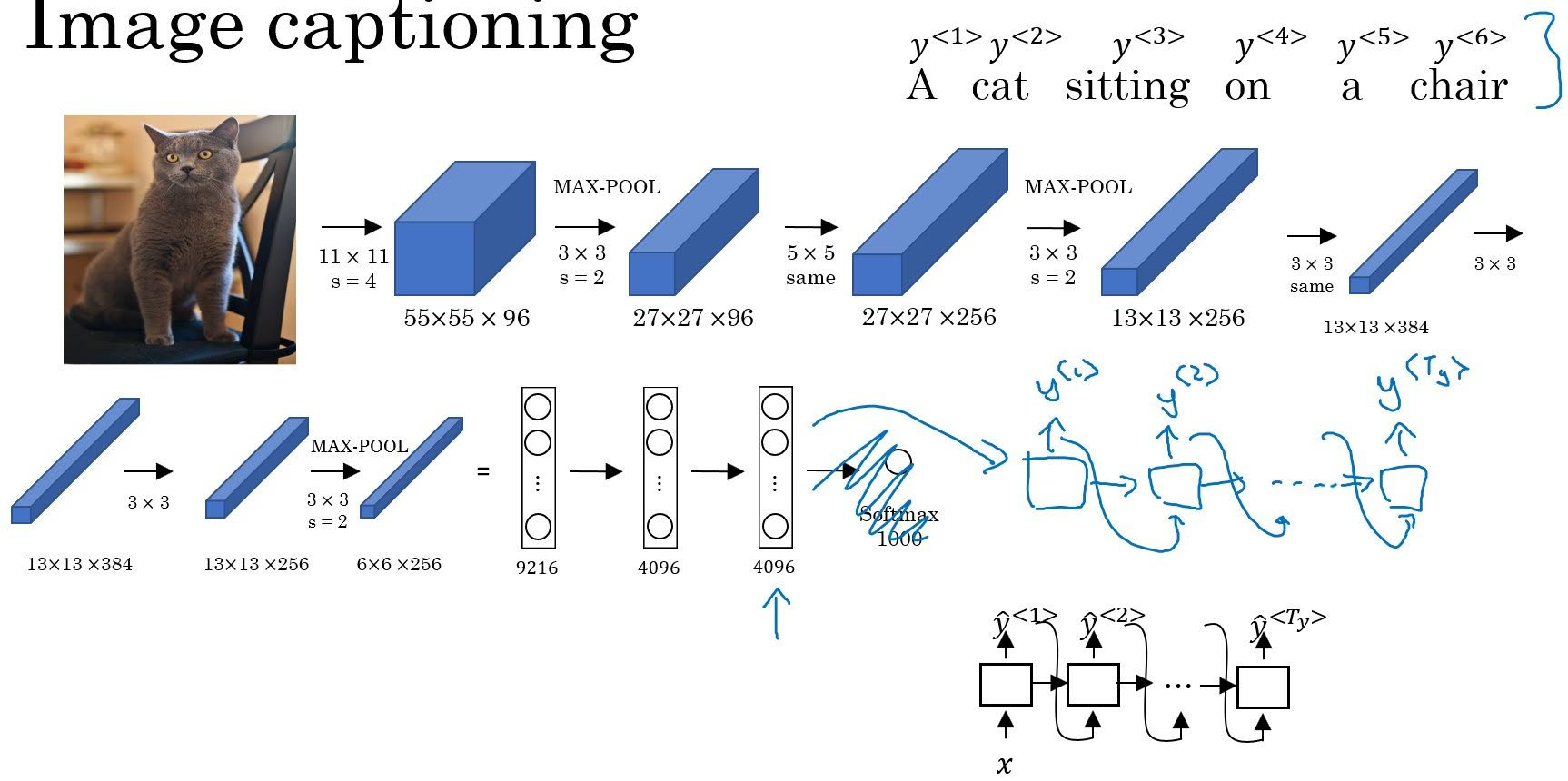# Sequence to Sequence Model

Categories:

Updated:

Sequence to sequence model is a many-to-many RNN architecture where we encode a sequence and decode it as a sequence.# Examples of Sequence Models

Machine Translation is an example of sequnce to sequnce models. First, you input original sentence to an RNN(maybe a BRNN with LSTM). Then feed the output as $x^{<1>}$ of the second RNN. In the second RNN, predicted word from $\hat{y}^{<t>}$ becomes $x^{<t+1>}$ like in language model.Image Captioning is another example of encoding-decoding model (similar to sequence to sequence model, precisely an image to sequence model). We first encode the image with CNN, then with the encoded vector as an input train a language model like RNN.# Difference from Language Model

Sequence to sequence model is similar to language model but has two differences.

First, sequence to sequence model has an ‘encoding’ part. In language model, we’ve initialized $x^{<1>}$ with 0 vector, whereas in sequence to sequence model, we use output of the encoding RNN as $x^{<1>}$ in the decoding RNN.

Second, sequence to sequence model picks the ‘most’ likely sentence. In language model, we’ve randomly picked a word from distribution of $\hat{y}^{<t>}$ to feed into the next sequential layer. However, in sequence to sequence model, we want to pick the most likely sentence. So our objective is as follows;

where $T_y$ is the length of the output sequence.

Categories: